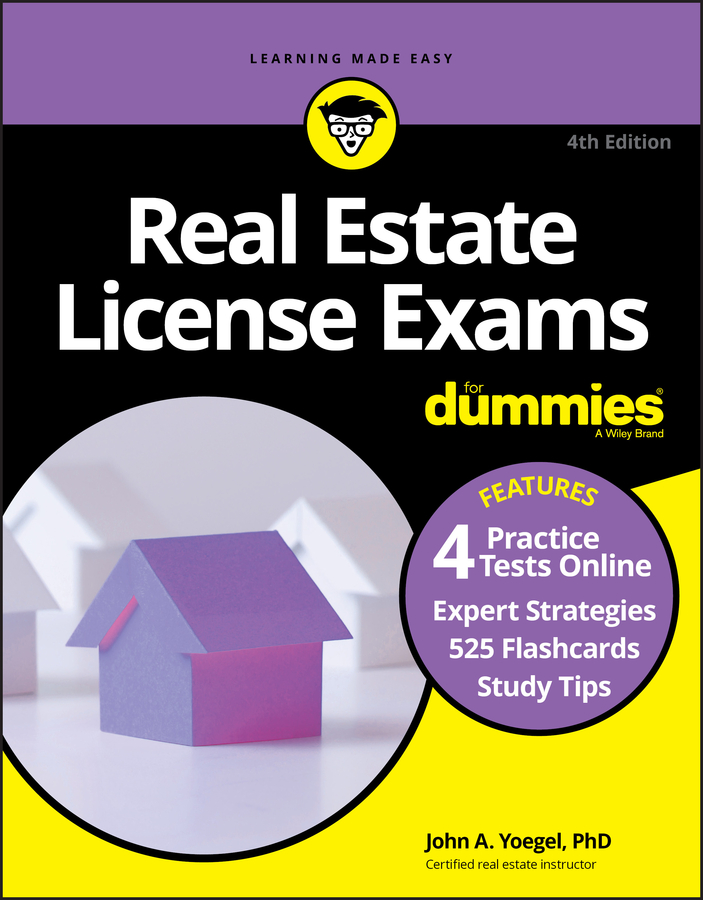##### Real Estate License Exams For Dummies with Online Practice TestsHere is a math problem you may see on the Real Estate License Exam. Establishing how much a house should sell for is an interesting calculation that has many uses, primarily because it enables you to work backward from a percentage to a number. But first, try this problem.

Your seller wants to net (take away from the closing table) \$200,000 after paying your 6 percent commission. How much does the house have to sell for to do this?

This problem actually has two answers, \$212,000 and \$212,766 (rounded), but one of the answers, \$212,000, happens to be wrong. Don’t feel bad that you got it wrong. Most people come up with the wrong answer. But by the time they do a few problems like the one that follows, nobody gets it wrong. This proof may come in handy on an exam to check your answer.

Selling price x commission rate = commission paid

\$212,000 x 0.06 = \$12,720

Selling price – commission paid = net to seller

\$212,000 – \$12,720 = \$199,280

The number \$199,280 obviously isn’t the \$200,000 net the seller wants. If you got \$212,000 for your answer, it’s because you added the 6 percent commission to the net that the seller wanted, but that isn’t how you calculate selling prices and commissions.

You get a commission by taking it from the selling price, not adding onto the net to the owner figure. If you did the same proof using the \$212,766 and took 6 percent from that number, the owner gets the requested \$200,000.

Check out the following example if you want more clarification:

What it is Percentage Dollar amount

Selling price 100 percent x (That’s what you’re looking for.)

Commission 6 percent You don’t know this and don’t need to know.

Net to seller 94 percent \$200,000

See how the commission percentage and the seller’s net percentage always equal 100 percent, which is the selling price.

If you know a dollar amount and it’s part of a larger dollar amount that you want to find, and you know the percentage of the total (100 percent) that it represents, you can always find the larger dollar amount by dividing the partial dollar amount by the percentage of the total that it represents.

\$200,000 is the dollar amount that is part of a larger dollar amount that you want to find — that is, the selling price. \$200,000 is 94 percent of the larger dollar amount, which is 100 percent. By dividing \$200,000 by 94 percent, you get the larger amount, which is 100 percent of the total.

\$200,000 ÷0.94 = \$212,766

In other words, if \$200,000 is 94 percent of some number that you want to find (the selling price, in this case), all you have to do is divide \$200,000 by 94 percent (0.94). You get \$212,766.

In this particular problem, you had to subtract 6 percent from 100 percent to get the 94 percent, which is the seller’s share. But the test writers may write the problem a little differently, trying to trick you.

You earn an \$18,000 commission, which is 6 percent of the selling price. How much did the house sell for?

Using the logic for this kind of problem, you can restate it like this: \$18,000 is 6 percent of some number. In this case, that number is the selling price. And if you know the dollar amount and the percentage of the whole number it represents, all you have to do is divide by the matching percentage.

\$18,000 (commission amount) ÷0.06 (commission rate) = \$300,000 (selling price)

So what’s the moral of the story? When figuring commissions in relation to how much a house should sell for, memorize these two formulas:

Net to seller ÷(100 percent – commission rate) = selling price

Commission amount in dollars ÷commission rate = selling price